###### Descriptions and Examples for the POV-Ray Raytracerby Friedrich A. Lohmüller     Elementary Geometry for Raytracing
Italiano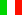Français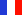Deutsch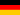Home
- POV-Ray Tutorial

- Geometrical Basics
for Raytracing

Right-angled Triangle
Pythagorean Theorem
Trigonometry Basics
Law of cosines
Equilateral Triangle
Regular Polygon
Polyhedron
Tetrahedron
Octahedron
Cube & Cuboid
Dodecahedron
Icosahedron
Cuboctahedron
Truncated Octahedron
Rhombicuboctahedron
Truncated Icosahedron
Circles
Tangent Circles
Internal Tangents
External Tangents

- Geometric 3D Animations

## Tangent circlesIn the following we write for the square root of a number the expression "sqrt(ZAHL)" conforming to the syntax used in POV-Ray.Note: Here objects in 2D geometry are represented by 3D shapes in the xy-plane. Therefore all coordinates must have the z-components zero! ( <?,?,0>)

 Circle 1: center M1 = <0.35,0,0>, radius r1= 0.22. Circle 2: center on y-axis, tangents Circle 1 from outside in the point S. Problem 1: Circle 2 has the radius r2 = 0.30 . Where on the y-axis (height y2) is the center of the second circle 2 ? (M2 = <0,?,0>) Problem 2: Circle 2 has the center M2 = <0,0.40,0>. How big must be the radius of a second circle 2, if it intersects (touches) the first circle 1 at the nearest point? Problem 3: What are the coordinates of the point S ? Problem 4: What are the angles at M1 and M2 inside the triangle(0,M1,M2)? The triangle(O,M1,M2) is rectangular! Therefore we use the Pythagorean Theorem: // Problem 1: //-------------------------- We know two sides of the triangle(O,M1,M2): d(0,M1) = x1 and d(M1,M2) = r1+r2.    y2 = sqrt( (r1+r2)2 - x12)    M2 = <0,y2,0>. // Problem 2: //-------------------------- Because of    r1+r2 = d(M1,M2) = sqrt(x12 + y22), we get    d(M1,M2) = sqrt( x12 - y22 )    and r2 = d(M1,M2) - r1. // Problem 3: //-------------------------- There is a simple proportionality:   xS1/xM1 = r2/ (r1+r2) and yS/yM2 = r1/ (r1+r2), so: xS = xM1 · r2/ (r1+r2)       yS = yM2 · r1/ (r1+r2). // Problem 4: //-------------------------- By the inverse trigonometric function i.e. of tan(x):    Angle(M1) = atan ( y2/ x1),    Angle(M2) = 90 - Angle(M1).Tangent circles rendered with POV-Ray
Note: To avoid any collision with built-in identifiers and reserved words in POV-Ray, it's strongly recommanded to use only words beginning with capital letters for all identifiers of variables declared by the user,
i.e. use "R1" instead of "r1" and use "Y2" instead of "yM2".
 ```#local R1= 0.22; #local R2= 0.30; #local M1 = <0.35,0,0> #local Y2 = sqrt( pow(R1+R2, 2) - pow(M1.x, 2)); #local M2 = <0,Y2,0>; ```
Problem 1 in POV-Ray
 ```#local R1= 0.22; #local M1 = <0.35,0,0> #local M2 = <0.40,0,0> #local R2 = sqrt( pow(M1.x,2)-pow(M2.y,2)) - R1;```
Problem 2 in POV-Ray
 ```#local XS = M1.x * R2/(R1+R2); #local YS = M2.y * R1/(R1+R2); #local S = #local Angle_M1 = degrees( atan( M2.y / M1.x) ); #local Angle_M2 = 90 - Angle_M1;```
Problem 3 + 4 in POV-Ray

For what can we use this geometry?
Here some examples:
We can consider the circle_1 as the cross-section of an horizontal torus and the circle_2 as the cross-section of a bowl:

We can consider the circle_1 and its twin mirrored at the y-axis as the cross-section of two cylinder in z-direction
and the circle_2 as the cross-section of another cylinder to subtract from the yellow rectangle: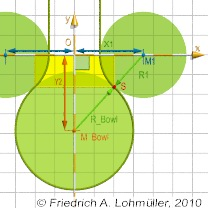Round-bottom Macefor the labratoryTwo cylinders melting together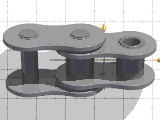Chain link for a bike chain
 © Friedrich A. Lohmüller, 2010 http://www.f-lohmueller.de